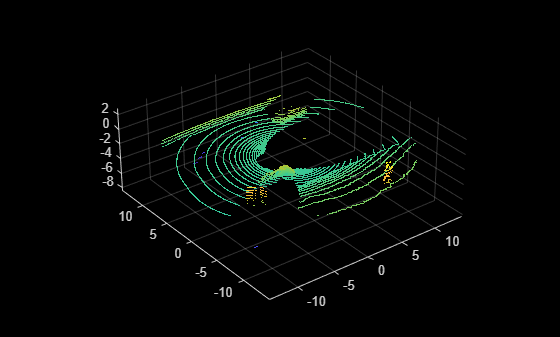# findPointsInCylinder

Find points within a cylindrical region in a point cloud

Since R2023a

## Syntax

``indices = findPointsInCylinder(ptCloud,radius)``
``indices = findPointsInCylinder(___,Name=Value)``

## Description

example

````indices = findPointsInCylinder(ptCloud,radius)` finds the points within a cylindrical neighborhood of the specified `radius` in a point cloud, ptCloud.```
````indices = findPointsInCylinder(___,Name=Value)` specifies options using one or more name-value arguments in addition to the previous syntax. For example, `Height=10`, sets the height of the cylinder to 10.```

## Examples

collapse all

Load a point cloud from a saved MAT file.

`load("drivingLidarPoints.mat","ptCloud")`

Specify a hollow cylindrical neighborhood with an inner radius of 2 meters and an outer radius of 15 meters, and find the points in the point cloud within the neighborhood.

```minRadius = 2; maxRadius = 15; indices = findPointsInCylinder(ptCloud,[minRadius maxRadius]);```

Select the cylindrical neighborhood in the point cloud, preserving the organized format.

`ptCloudCylinder = select(ptCloud,indices,OutputSize="full");`

Visualize the selected points.

```figure pcshow(ptCloudCylinder)```Load a point cloud from a saved MAT file.

```load("object3d.mat","ptCloud") maxRadius = 0.1; cylinderHeight = 0.5;```

Offset the center of the cylinder 0.6 units in the X-direction and 0.2 units in the Z-direction. Then, find and select the points of the point cloud within the cylinder.

```shiftedCenter = [0.6 0 0.2]; indices = findPointsInCylinder(ptCloud,maxRadius,Height=cylinderHeight,Center=shiftedCenter,VerticalAxis="Z"); ptCloudCylinder = select(ptCloud,indices);```

Visualize the selected points.

```figure pcshow(ptCloudCylinder)```## Input Arguments

collapse all

Point cloud, specified as a `pointCloud` object.

Cylinder radius, specified as a scalar or a 2-element vector of the form [minRadius maxRadius] that specifies the inner and outer radii of the cylindrical neighborhood. The function finds the neighbors within the specified `radius` around a query point in the input point cloud.

### Name-Value Arguments

Specify optional pairs of arguments as `Name1=Value1,...,NameN=ValueN`, where `Name` is the argument name and `Value` is the corresponding value. Name-value arguments must appear after other arguments, but the order of the pairs does not matter.

Example: `Height=10` sets the height of the cylinder to 10.

Height of cylinder, specified as a positive scalar.

Center of the cylinder, specified as a 3-element vector of the form [x y z] in Cartesian coordinates. The center point is halfway between the top and bottom of the cylinder, and the axis of the cylinder passes through it.Vertical axis of the cylinder, specified as `"X"`, `"Y"`, or `"Z"`.

## Output Arguments

collapse all

Logical indices of the points within a cylindrical neighborhood, returned as a column vector for unorganized point clouds or a matrix for organized point clouds. For organized point clouds. `indices` is the same size as the input point cloud, and each element with a value of `1` (true) indicates that the corresponding index of the input point cloud references a point inside the specified cylindrical neighborhood.

## Version History

Introduced in R2023a